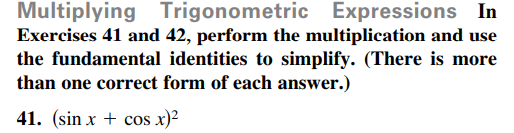### Still have math questions?Multiplying Trigonometric Expressions In Exercises $$4 1$$ and $$4 2$$ , perform the multiplication and use the fundamental identities to simplify. (There is more than one correct form of each answer.) 41. $$( \sin x + \cos x ) ^ { 2 }$$
$$(\sin x+ \cos x)^2 = 1+ \sin(2x)$$